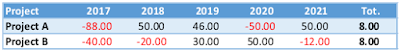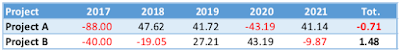## Wednesday, May 17, 2017

### 3 KPIs to Select a Project

In the last two posts, we talked about the utilization of KPIs in project management, identifying 10 simple KPIs, useful to keep a project under control from different perspectives.

In the present post, we will identify indicators, useful to select which projects in a portfolio are more worth to be executed.

Before diving into the world of indicators, we have to make a quick clarification.
It is common wisdom that one dollar today worths more than a dollar tomorrow. As a matter of fact, money’s values is affected by material factors, like inflation or borrowing interest rates, and by perceptional factors, like uncertainty related to collecting money.
Since in project management is quite common compare projects that span many years, with different cash flow statements, it is evident the necessity to have a method that makes possible compare cash flows and figures registered at various times. An actualization of numbers is the only fair way to compare different projects.
Fortunately, the needed method exists, it is called discounted cash flow and its mathematical formalization is quite straightforward.

Let’s assume we need to compare the value of 10 dollars today, with the value of 15 dollars three years from now, knowing that inflation rate is fixed at 5%. We can proceed in two ways, bringing the value of 10 dollars today in the future, or actualizing the value of 15 dollars three years from now.

Bringing the value of 10 dollars today, three years  in the future, considering a 5% fixed inflation rate

Actualizing the value of 15 dollars three years from now, considering a 5% fixed inflation rate

Having said that, please remember that, in the rest of the post, we will always refer to actualized values, when talking about figures and cash flows.

For your convenience, Figure 1 is a conversion lookup table that depicts how much is worth a dollar today, imagining a fixed discount rate (first column) and a given number of years (first row).Figure1. Conversion lookup table. Rows are discount rates. Columns are years.

## Net Present Value

You may have heard the adage “Cash is king!”, talking about a company’s balance sheet. It is almost the same about projects. When it comes to making a decision, an analysis of the cash flow generated by a project is almost always a good approach.
The purpose of net present value is to compare projects that accrue different expenditures and incomes in different times. The index is simply an algebraic summation of all the incomes/expenditures of a project for each year, actualized at a given time, using the discounted cash flow formula. In the following equation, C represents the cash flow accrued in year i, r the applied discount rate, and i the current year.

Let’s try a simple example, for the sake of clarity. We consider two projects which different cash flows, accrued in different times. Which of the two projects, making a decision based just on the net cash flow, would be more worth to be executed?
The foreseen cash flows generated by two projects are reported in Table 1, in thousands of Euros. From the presented view, it seems that both projects generate the same total amount of cash flow, even if displaced in time.Table 1.

The actualization process will be performed on an annual basis, concerning 2017, considering a hypothetical, fixed discount rate of 5%. Remember, it is just an example. The rationale still holds if you do the math on a monthly basis, or if you apply compound and time-variable rates.
The actualized figures of the two projects are reported in Table 2. As we can see, Project B is preferable, since it is the only one that yields a positive return.Table 2.

If you rely on the net present value as an indicator to select a project, choose the project with the highest value. Obviously, you cannot rely just on net present value to compose a sound portfolio of projects, still, is definitely an indicator to take in consideration.

## Return on Investment

The first thing to know: it is return on investment and not return of investment.
The purpose of return on investment is to measure and evaluate the efficiency of one or more investments, taking into account returns and costs. What is a project, if not another kind of investment, which accrues costs and (hopefully) revenues?
The formula is a percentage, expressed as

The percentage form is incredibly useful to compare different projects easily.
Even in this case, revenues and costs shall be actualized using the discounted cash flow formula.
Applying the return on investment to the project described in the previous example, we obtain a -0.5% ROI for project A and a 2.15% ROI for project B.
If you rely on the return on investment as an indicator to select a project, choose the project with the highest value.
Again, my advice is not to rely on a single index, if you want to increase the opportunity to build a sound portfolio of projects.

## Internal Rate of Return

The Internal rate of return is quite tricky an index to understand; at least, for me, it has always been.
Mathematically speaking, the internal rate of return is simply the discount rate that puts to 0 the value of the net present value of a project. So, to evaluate the IRR, you must solve the following equation for r (I know, it is not simple)

To simplify, think about the internal rate of return as the “velocity” with which the project will earn returns. The higher the index, the faster the company will receive the value provided by the project.
So, even for the internal rate of return, the higher the value, the better.
It is quite reasonable to use the internal rate of return as a companion for the net present value. The net present value shows how much the project will earn (or lose), the internal rate of return shows how fast the expected outcomes will be materialized.Quest' opera è distribuita con licenza Creative Commons Attribuzione - Non commerciale - Non opere derivate 3.0 Unported.